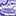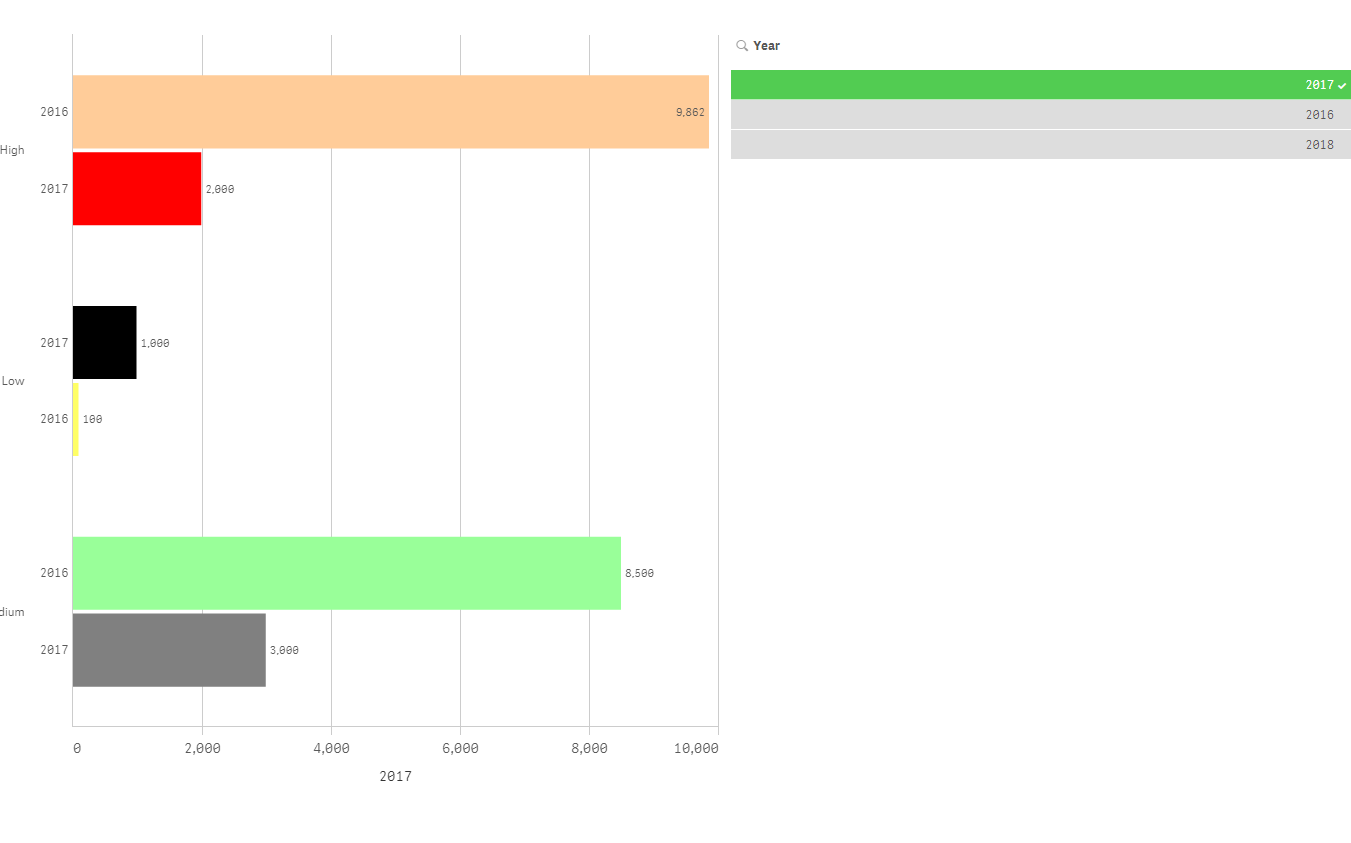# New to Qlik Sense

If you’re new to Qlik Sense, start with this Discussion Board and get up-to-speed quickly.

Announcements
Qlik Cloud Maintenance is scheduled between March 27-30. Visit Qlik Cloud Status page for more details.
cancel
Showing results for
Did you mean:Creator II

## Bar chart color coding help

Hi Experts,

I have applied below color expression in the bar chart for all current year high, low and medium need to show Red, black and grey.

for all previous year high, low and medium need to show orange, yellow and green.

=if(Category='Low' and Year=\$(=Max(Year)),RGB(0,0,0),

if(Category='Low' and Year=\$(=Max(Year)-1),RGB(255,255,102),

if(Category='High' and Year=\$(=Max(Year)),RGB(255,0,0),

if(Category='High' and Year=\$(=Max(Year)-1),RGB(255,204,153),

if(Category='Medium' and Year=\$(=Max(Year)),RGB(128,128,128),

if(Category='Medium' and Year=\$(=Max(Year)-1),RGB(153,255,153)))))))

But when I have selected 2017 then previous year 2016 color coding not working. Please help me on this.

1 Solution

Accepted SolutionsMVP

Try this as your expression for color

=Only({1}if(Category='Low' and Year=\$(=Max(Year)),RGB(0,0,0),

if(Category='Low' and Year=\$(=Max(Year)-1),RGB(255,255,102),

if(Category='High' and Year=\$(=Max(Year)),RGB(255,0,0),

if(Category='High' and Year=\$(=Max(Year)-1),RGB(255,204,153),

if(Category='Medium' and Year=\$(=Max(Year)),RGB(128,128,128),

if(Category='Medium' and Year=\$(=Max(Year)-1),RGB(153,255,153))))))))MVP

Try this as your expression for color

=Only({1}if(Category='Low' and Year=\$(=Max(Year)),RGB(0,0,0),

if(Category='Low' and Year=\$(=Max(Year)-1),RGB(255,255,102),

if(Category='High' and Year=\$(=Max(Year)),RGB(255,0,0),

if(Category='High' and Year=\$(=Max(Year)-1),RGB(255,204,153),

if(Category='Medium' and Year=\$(=Max(Year)),RGB(128,128,128),

if(Category='Medium' and Year=\$(=Max(Year)-1),RGB(153,255,153))))))))Tags
Community Browser